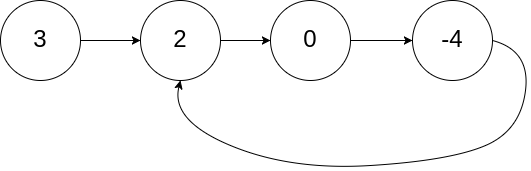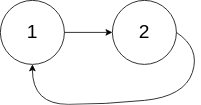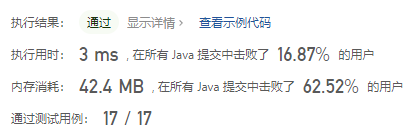#### 142. 环形链表 II

###### 来源: 每日一题 2023.07.30``````输入：head = [3,2,0,-4], pos = 1

````````````输入：head = [1,2], pos = 0

````````````输入：head = , pos = -1

``````

• `链表中节点的数目范围是 [0, 10^4]`
• `-10^5 <= Node.val <= 10^5`
• `pos 为 -1 或者链表中的一个 有效索引 。`
``````/**
* public class ListNode {
*     int val;
*     ListNode next;
*     ListNode(int x) { val = x; }
* }
*/
class Solution {

}
}
``````

#### 分析与题解

• 缓存暴力法

一个链表是否有环, 在昨天已经实现了, 我们是利用了两个快慢指针来判别是否有环, 当我们需要判别环的起始点时, 我们可以利用缓存存储我们遍历过的点, 当点再次出现的时候就是我们需要的题解.

• 首先,需要做结果的边界处理.

``````if (head == null) {
return null;
}
``````
• 然后就是遍历, 并且查找起始点.

``````Set<ListNode> cache = new HashSet<ListNode>();
ListNode result = null;
break;
}
}
``````

整个解题过程如下所示.

``````/**
* class ListNode {
*     int val;
*     ListNode next;
*     ListNode(int x) {
*         val = x;
*         next = null;
*     }
* }
*/
public class Solution {
return null;
}
Set<ListNode> cache = new HashSet<ListNode>();
ListNode result = null;
break;
}
}
return result;
}
}
``````

复杂度分析:

• 时间复杂度: O(n)
• 空间复杂度: O(n)

结果如下所示.IT界无底坑洞栋主 欢迎加Q骚扰:676758285